When creating prediction calculations using the predictive modelling functions in Tableau, you’ll need to choose predictors. As a reminder, a predictor is an input variable whose value is used to predict an outcome variable, also known as the target or response. Often, you’ll be working with data you have extensive domain knowledge of and will already have a good sense of which fields are strongly correlated with your prediction target, and would make good predictors. However, it's still a good idea to take the time to evaluate your predictors and ensure that you're choosing them wisely. You will always want to include at least one predictor, and usually more than one.

First, select your target. This sounds obvious, but making sure that you're selecting your predictors based on what you want to predict is a critical first step. For example, the fields that are most correlated with female life expectancy might be very different from the fields most correlated with male life expectancy. Similarly, the fields most correlated with sales could be very different from the fields most correlated with profit.

Another thing to keep in mind is that by default, the predictive modelling functions use linear regression as the underlying statistical model. With this model, the most correlated predictors are those that have a linear relationship with the target. For information on using another supported model, see Choosing a Predictive Model.

To better understand how to choose the best predictors for the questions you’re aiming to answer, let’s look at female life expectancy data. To follow along, download the following workbook from Tableau Public: Choosing Predictors for Your Predictions.

## Measures as predictors

When using a measure as a predictor, you can evaluate its correlation with your target using Tableau. One way is to build a scatter plot. Below, we compare a country’s median female life expectancy with a variety of other measures.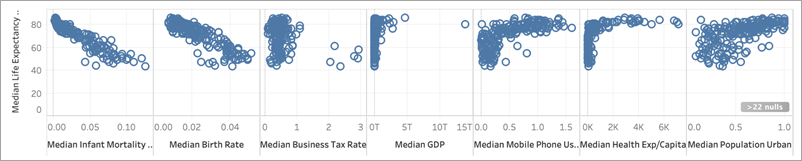For some measures, like Infant Mortality and Birth Rate, there's a clear negative correlation with Female Life Expectancy, as seen in the negative slope of the scatter plot. For others, it's less clear. However, one thing that we can clearly see is an L-shaped distribution for Median GDP, Median Mobile Phone Usage and Median Health Exp/Capita. This L-shaped distribution often indicates that using a log transformation can help you analyse your data more accurately. Another clue is if all a column’s values are positive. In Tableau, you can use a log transformation by editing the pill and wrapping the expression in a LOG function: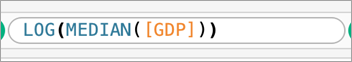This takes us from the L-shaped distribution – where it's hard to differentiate between the extremes of the scale – to a more even distribution less compressed by the extremes of the scale.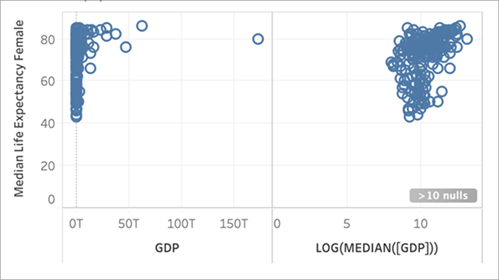Repeating this with the other L-shaped distributions gives us the following: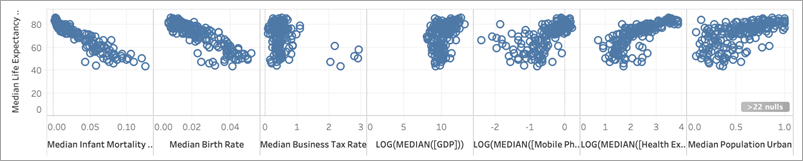### Coefficient of determination, or R-squared value

The closer the marks are to lying on a straight line, the higher the correlation between the two measures. To help evaluate the correlation, you can add trend lines. From the Analytics pane, drag Trend Line into the view and drop it on Linear. Hovering your cursor over the trend line will tell you the R-squared value, or coefficient of determination, which indicates how much of the dependent variable (the target) is explained by the independent variable (the predictor). Predictors with R-squared values closer to 1 are better than predictors with R-squared values closer to 0.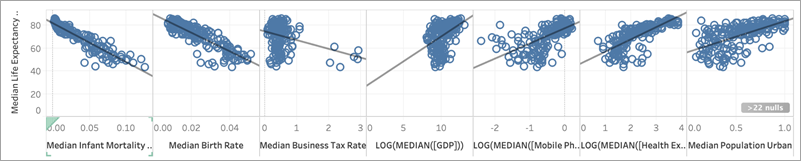Looking at our scatter plots, we can see that the best predictor for median female life expectancy is median infant mortality, which has an R-squared value of 0.87: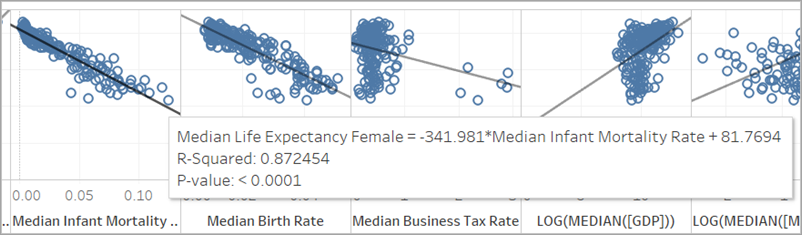Other good predictors are Median Birth Rate (R-squared=0.76) and the log transformation of Median Health Expenditure/Capita (R-squared=0.56).

Note: The slope of the line does not clearly show you which predictors have the highest R-squared value. Since the scale of the x-axis is determined by the range of the specific variable selected, the slope of the line is greatly affected by the specific variables used.

In the below image, we've visualised Median Female Life Expectancy against Median Female Life Expectancy, with the resulting viz being a perfectly straight line on a 45° angle: as expected, there is a perfect correlation between the value on the x-axis and the value on the y-axis, with an R-squared value of 1: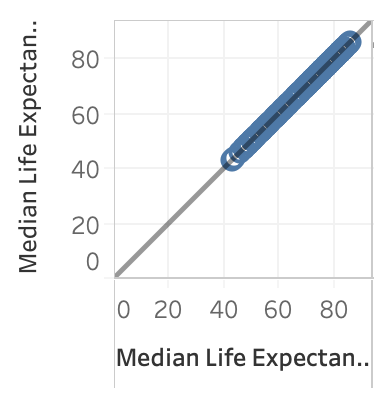However, as shown below, even though LOG(MEDIAN([GDP])) has a steeper sloped trend line than the others, it has a low R-squared score of only 0.169. This is due to the scale of the x-axis for that pane: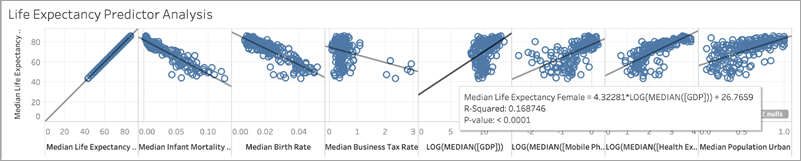Furthermore, let's take a look at how a few marks can significantly affect the slope of a trend line. Zooming in on the scatter plot for Median Business Tax Rate we can see that the majority of the marks have a tax rate between 0 and about 1, with six countries having much higher rates, between 2 and 3. The R-squared value for all marks is 0.0879: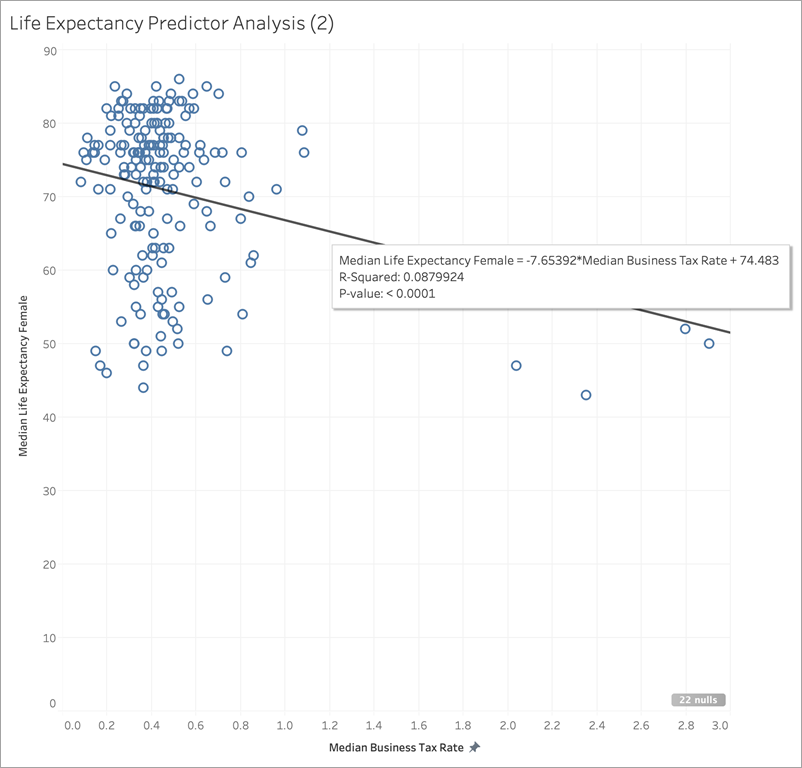However, let's see what happens if we remove that cluster of six marks: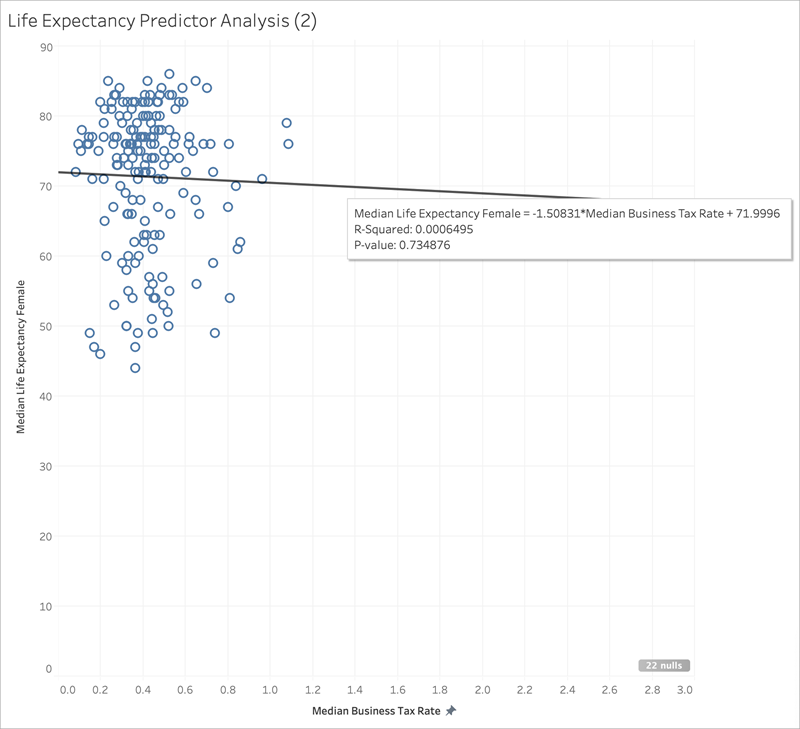The trend line goes nearly flat, and the R-squared value drops to 0.0006 – indicating that there's essentially no correlation between Median Business Tax Rate and Median Female Life Expectancy. As you visualise your data and use good statistical methods to select your predictors, it's important to carefully consider whether there are any outliers or other data characteristics that could affect your conclusions.

Note: For more examples of how summary statistics might not give you the full picture, read up on Anscombe's quartet.

## Dimensions as predictors

When using dimensions as predictors, you can use a similar procedure to determine correlation. However, you may find that there’s a significant discrepancy between different dimensions in their level of relationship to the target. For example, when subdividing by Region, one region may be a very good predictor for the target, but another region may have significantly less correlation. This doesn’t mean that you shouldn't use that dimension as a predictor, but you may want to consider whether using additional measures or dimensions will help improve your model, and in turn, your predictions.

Since we've determined the best predictors for our data set are Median Infant Mortality, Median Birth Rate, and the log transformation of Median Health Expenditure/Capita, let’s limit the viz to these three variables: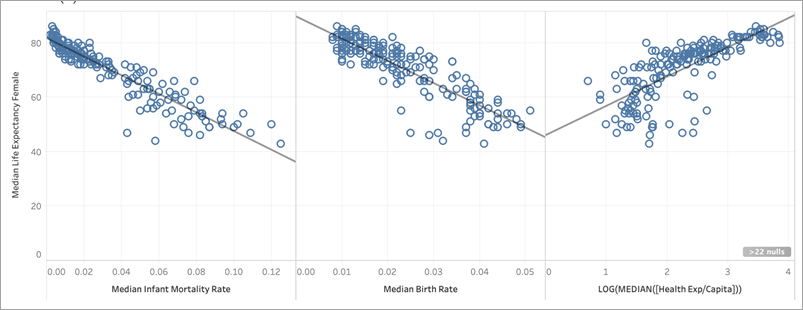Next, let’s partition the data by adding Region to Colour on the Marks card and see what happens to the viz: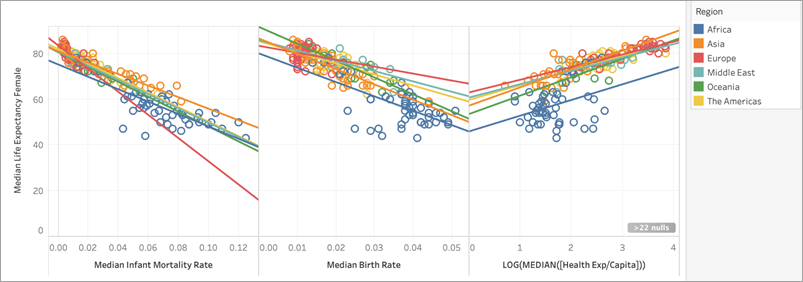### Comparing R-squared values across predictors

Let's see how the R-squared values compare for each of the Region trend lines for each predictor: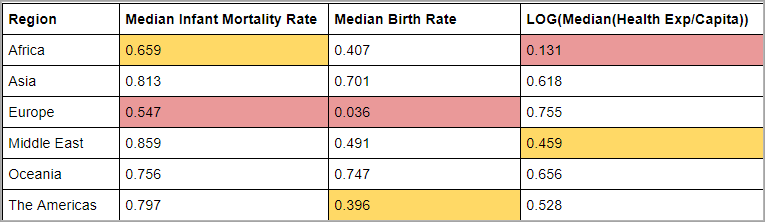In the table above, the lowest R-squared value for each predictor is highlighted in red, and the second-lowest is in yellow.

Europe has the lowest R-squared values for Median Infant Mortality and Median Birth Rate and Africa has the lowest R-squared value for the log transformation of Median Health Expenditure/Capita (highlighted in red). Africa also has lower R-squared values for both Median Infant Mortality Rate and Median Birth Rate.

Adding a dimension can provide your model with more information, and adding more information can improve the quality of your prediction. However, within a given subdivision (a Region, in this case), the prediction quality could either improve or decline. In some cases, you may want to build an individual model for each subdivision based on the measures that are the best predictors for that specific group.

In this case, Infant Mortality has a reasonably strong correlation with Female Life Expectancy for all regions, although it's somewhat weaker in Africa and Europe; Median Birth Rate is a good predictor for Oceania and Asia, but has almost no correlation with Female Life Expectancy in Europe, and the log transformation of Median Health Expenditure is a reasonable predictor for all regions except Africa. We can expect that the model built with all four predictors (Infant Mortality, Birth Rate, Log(Health Expenditure) and Region) will have the least accurate predictions for countries in Europe and Africa; we may want to do some deeper dives into the data to see if there are additional or alternative predictors we could use to build models that are better fits for Europe and Africa.

## Building your predictive modelling function

Now that we've found good predictors, we can build and apply a predictive modelling function to see it in action.

1. Open the Analysis menu at the top, and then select Create Calculated Field.

2. In the Calculation Editor, name the calculation, do the following:

• Name the calculation: Quantile_LifeExpFemale_HealthExpend,BirthRate,Mortality,Region

• Enter the following formula:

```MODEL_QUANTILE(0.5,MEDIAN([Life Expectancy Female]), LOG(MEDIAN([Health Exp/Capita])), MEDIAN([Birth Rate]), MEDIAN([Infant Mortality Rate]), ATTR([Region])) ```

This calculation will return the median value (0.5) of the range of modelled median female life expectancies, based on the predictors we selected: Health Expenditure, Birth Rate, Infant Mortality and Region.

Next, let’s build a scatter plot that shows both the actual median female life expectancy and the predicted median female life expectancy: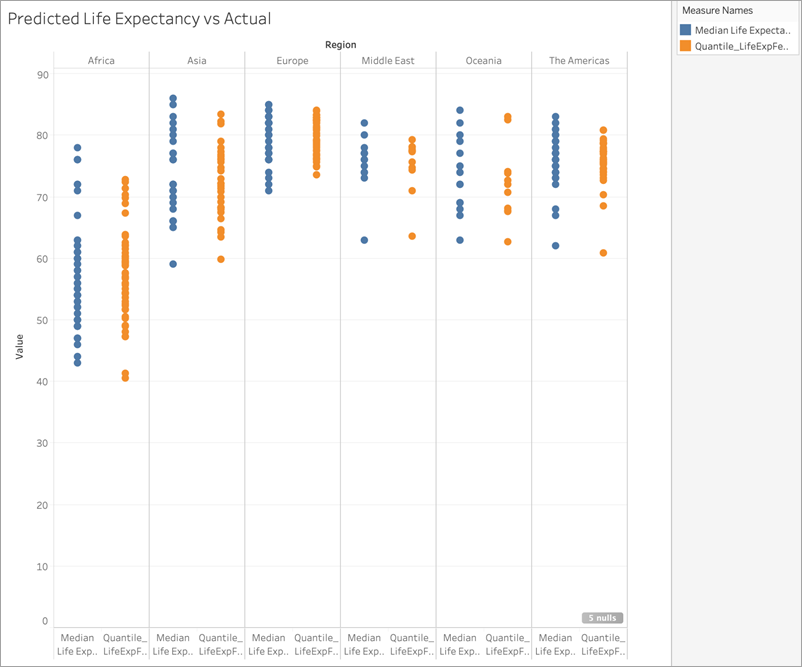Great! The predictions are pretty much in line with the actual values for each region.

But let's do another pass to figure out where the predictions are furthest off the mark. Create another calculation called Residual_LifeExpFemale_HealthExpend,BirthRate,Mortality,Region, as follows:

```MEDIAN([Life Expectancy Female]) - [Quantile_LifeExpFemale_HealthExpend,BirthRate,Mortality,Region] ```

This residual calculation will return the difference between the predicted median and the actual median, helping us see the countries where there's the biggest discrepancy between the actual and predicted median female life expectancy.

Next, let’s apply this residual calculation to Colour: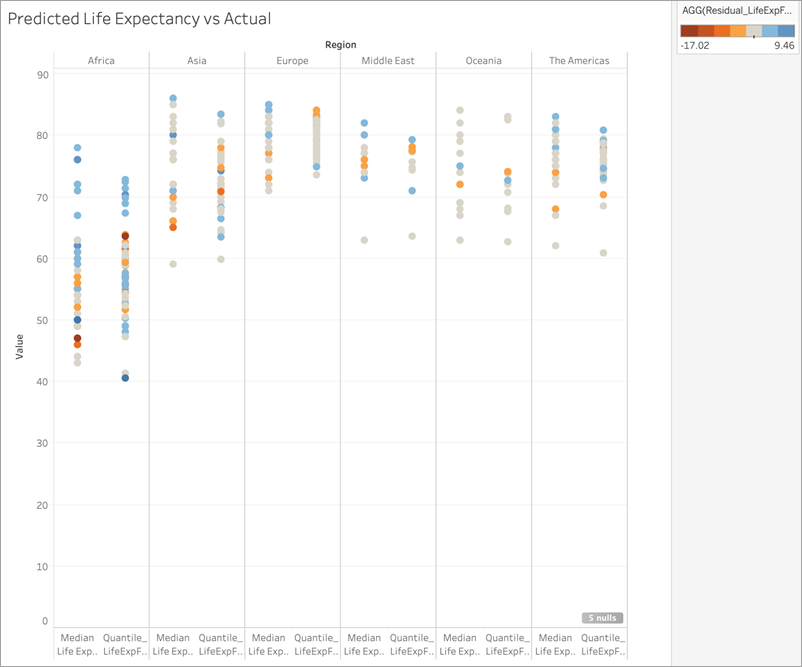You can see in the above viz that most countries, across most regions, have low discrepancies between predictions and actual values. Africa is the region with the largest number of countries with significant discrepancies, but let's do one more round to see what kind of differences we're looking at.

You can see that the differences range between -17 and +9, so let's divide the viz into groups where there's less than ±3 years difference, less than ±5 years difference, less than ±10 years difference and more than ±10 years difference.

Create one more calculation, called Grouped_Residual_LifeExpFemale_HealthExpend,BirthRate,Mortality,Region, as follows:

```IF [Residual_LifeExpFemale_HealthExpend,BirthRate,Mortality,Region] <= 3 AND [Residual_LifeExpFemale_HealthExpend,BirthRate,Mortality,Region] >= -3THEN "±3"ELSEIF [Residual_LifeExpFemale_HealthExpend,BirthRate,Mortality,Region] <= 5 AND [Residual_LifeExpFemale_HealthExpend,BirthRate,Mortality,Region] >= -5 THEN "±5"ELSEIF [Residual_LifeExpFemale_HealthExpend,BirthRate,Mortality,Region] <= 10 AND [Residual_LifeExpFemale_HealthExpend,BirthRate,Mortality,Region] >= -10 THEN "±10"ELSE "> ±10" END```

Again, let's add the calculation to Colour: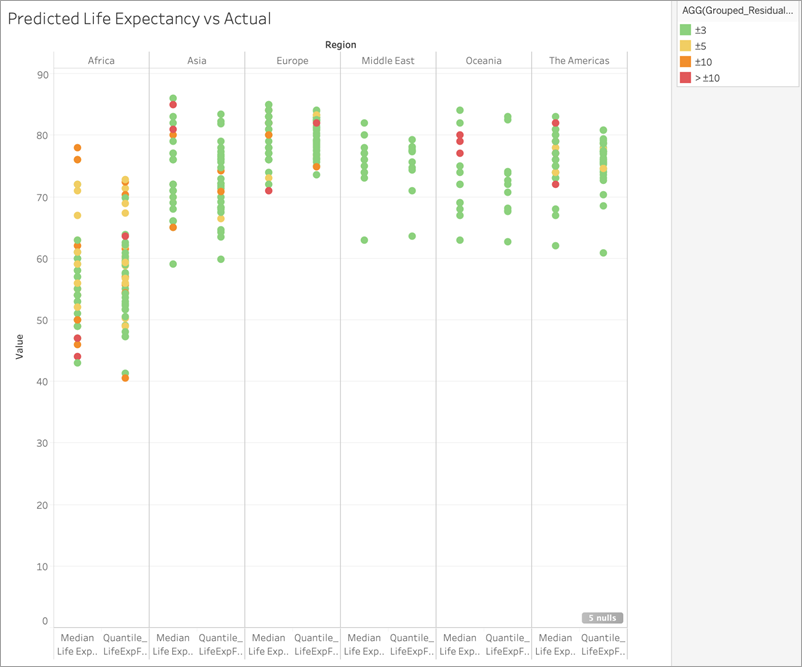Notice that the vast majority of predictions are incorrect by less than 3 years, and that only a small handful are off by more than 10 years. Overall, pretty good!

This means that using this model would allow us to accurately identify those countries with median female life expectancies that are outliers, or to provide the modelled female life expectancy for a country where this data was missing.